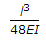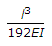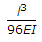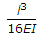# Civil Engineering - UPSC Civil Service Exam Questions - Discussion

17.

A simply supported beam of span l and flexural rigidity EI, carries a unit point load at its centre. The strain energy in the beam due to bending is

 [A].[B].[C].[D].Explanation:

No answer description available for this question.

 Shashideep Kumar said: (Jul 22, 2017) Please give the explanation of the solution.

 Soumik said: (Feb 14, 2018) I think option A is correct. Yc=wl^3/48 EI for simply supported beam with point load on its centre.

 Mohit Singh said: (Mar 15, 2018) since strain energy due external load = 0.5 x W x deflection. And deflection for that beam you know.

 Yasir Ashrafi said: (Aug 17, 2018) The correct Answer must be A.

 Jerish David said: (Sep 3, 2018) Correct answer is option C only. U= Integral(M^2*ds/2EI). M= Wl/4, Where, W = 1(unit load), Substitute and integrate, You get, U = (W^2*l^3)/(96EI), U = l^3/96EI.

 Musthafa said: (Sep 6, 2018) u=.5 * w * deflection. w=1, put deflection will get option C.

 Kripa said: (Sep 30, 2019) U = 0.5*P*(PL^3)/96EI. P = Load (unit load here). U = L^3/96EI.

 Deepinder Singh said: (Jul 10, 2020) The formula is = integral (M^2 dx/2EI). M= WL/4 (as we know point load is given at the centre then the maximum bending moment is wl/4). W= 1 (Unit load assume this value). Substitute and Integrate using the formula: You will get = (W^2 X L^3)/(96 EI). Therefore the answer is = (L^3)/96EI.